output.to from Sideway
Number Theory

Factorization

Pollard's p-1 Method

Draft for Information Only

# Content

``` Pollard's p-1 Method     Pollard's P-1 Methed by PowerSmooth Number     Pollard's P-1 Methed by PowerSmooth Number Example 2```

# Pollard's p-1 Method

Pollard's p-1 method is a prime factorization algorithm discovered by John Pollard in 1974. Limited by the algorithm, the Pollard's p-1 method is only work for integers with specific factors.

If the number n has a prime factor p such that p-1 can be expressed in terms of a product of primes, the finding of prime factor p is based on the selected boundary B of the B-powersmooth number.  The algorithm fails when the selected boundary B is smaller than the B-powersmooth number of p-1.

#### Pollard's P-1 Methed by PowerSmooth Number

##### Pollard's P-1 Methed by PowerSmooth Number Example 2

For example: n=667=p*q=23*29; let B=5 imply

Integer B-smooth number Prime Factors number
k 5 22*31*51 = 4*3*5 60

Therefore for B=5, k5 or (p5-1)m5 is equal to 60.

###### Fermat's Little Theorem

let a=2, by Fermat's little theorem, let p be one of the prime factors of n, imply p idivides ak-1.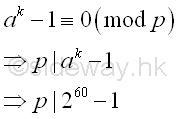###### Greatest Common Divisor

Since ak-1 is a very large number, before finding the greatest common divisor of n and ak-1,  ak-1 can be raised to the high power modulo n. Imply

Using squarings modulo

base  number; a=2; k=60; n=667
ak base 10 260
ai base 10 21  = 21 ≡ 2 (mod 667)
22 = 22 ≡ 4 (mod 667)
24 = 42 ≡ 16 (mod 667)
28 = 162 ≡ 256 (mod 667)
216 = 2562 ≡ 170 (mod 667)
232 = 1702 ≡ 219 (mod 667)
ak base 10 232+16+8+4
ak base 10 232*216*28*24
ak base 10 219*170*256*16 ≡ 538 (mod 667)

and using the residue to calculate the greatest common divisor. Imply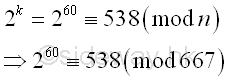The greatest common divisor of n and ak-1 is

Using Euclid's algorithm

ak-1 n
260-1 667
538-1 667
537 667-537=130
537-4*130=17 130
17 130-7*17=11
17-11=6 11
6 11-6=5
6-5=1 5
1 5-5*1=0
1 0

Imply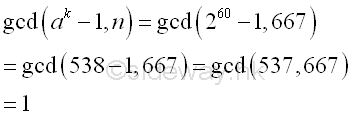The algorithm fails because the greatest common divisor of n and ak-1 is equal to 1. The number n does not have prime divisor p with p-1 is 5-powersmooth. Therefore the bound B equals to 5 fails, a larger bound B' should be used.

Let B=7,  imply

Integer B-powersmooth number Prime Factors number
k 22*31*51*71 = 4*3*5*7 60 * 7 = 420

Therefore for B=7, k7 or (p7-1)m7 is equal to 60*7 = 420. Imply  k7 =  k5 * 7. And  the new ak can be expressed as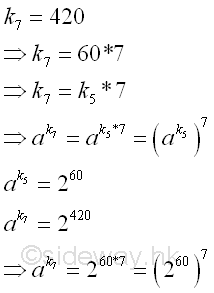And ak can be raised to the high power modulo n, imply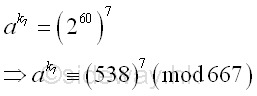Using squarings modulo

base  number; a=538; k=7*; n=667
ak base 10 5387 (mod 667)
ai base 10 5381  = 5381 ≡ 538 (mod 667)
5382 = 5382 ≡ 633 (mod 667)
5384 = 6332 ≡ 489 (mod 667)
ak base 10 5384+2+1 (mod 667)
ak base 10 5384*5382*5381 (mod 667)
ak base 10 489*633*538 ≡ 349 (mod 667)

and using the residue to calculate the greatest common divisor. Imply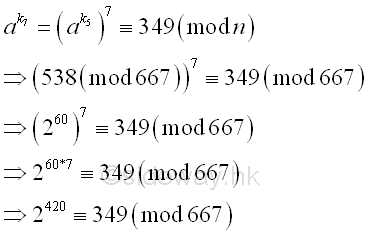The greatest common divisor of n and ak-1 is

Using Euclid's algorithm

ak-1 n
2420-1 667
349-1 667
348 667-348=319
348-319=29 319
29 319-11*29=0
29 0

Imply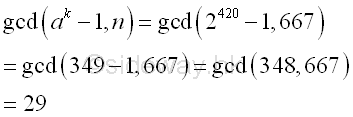Integer 29, the greatest common divisor of n and ak-1 is also the prime divisor of n. And p-1 is  7-powersmooth.

Integer B-powersmooth number Prime Factors number
p-1 7 22*71 28
k 7 22*31*51*71 = 4*3*5*7 420
k/(p-1)   20*31*51*70 15

ID: 120500010 Last Updated: 17/5/2012 Revision: 0Home 5

Management

HBR 3

Information

Recreation

Culture

Chinese 1097

English 337

Computer

Hardware 149

Software

Application 187

Numeric 19

Programming

Web 757

CSS 1

HTML

Knowledge Base

OS 389

MS Windows

Knowledge

Mathematics

Algebra 20

Number Theory 206

Geometry 18

Calculus 67

Engineering

Mechanical

Rigid Bodies

Statics 92

Dynamics 37

Control

Physics

Electric 10